# When Does A Limit Not Exist? (4 Key Cases To Know)

When working with functions in calculus, we will sometimes find that a limit does not exist.  There are lots of reasons this can happen, and it helps to be familiar with each one so you know what to look for.

So, when does a limit not exist?  The limit of a function at a point does not exist in 4 cases: 1. when the left hand limit does not exist, 2. when the right hand limit does not exist, 3. when the left and right hand limits exist, but have different values, and 4. when the function value is undefined, due to a domain restriction.

Of course, the existence of limits for a function can also tell us about continuity and differentiability of the function at certain points.

In this article, we’ll talk about when a limit does not exist.  We’ll look at some examples of each case to make the concept clear.

Let’s get started.

Having math trouble?

Looking for a tutor?

## When Does A Limit Not Exist?

A limit does not exist in the following cases:

• Left Hand Limit Does Not Exist
• Right Hand Limit Does Not Exist
• Left & Right Hand Limits Both Exist, But They Have Different Values
• Function Is Not Defined Due To Domain Restriction

Note that there are a few ways for a left or right hand limit to not exist, including:

• Oscillating values of the limit
• Unbounded increase or decrease (a vertical asymptote, or a limit of infinity)

Let’s start by looking at cases and examples where the left hand limit does not exist.

### Left Hand Limit Does Not Exist

In order for a limit to exist, both the left and right hand limits must exist, and they must have the same value.  Here are some examples where the left hand limit does not exist.

#### Example 1: Left Hand Limit Does Not Exist (Oscillating Values)

Consider the function f(x) = sin(1 / x2).  If we take a left hand limit as x approaches zero:

• Limx->0-f(x)

we will find that the limit does not exist.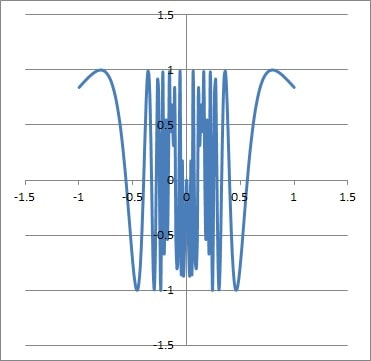The graph of the function f(x) = sin(1 / x2) near x = 0. It oscillates from -1 to 1 and does not settle down to a single value.

This is because the sine function oscillates between all values from -1 to 1 as x increases.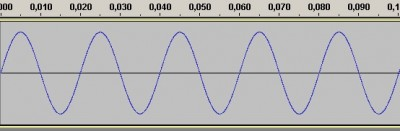The sine function continues to oscillate as x increases. It never settles down to a single value.

As x approaches zero, 1 / x2 increases without bound.  So, the limit will never “settle down” to a single value, and will continue oscillating forever.

Thus, the left hand limit does not exist in this case.

#### Example 2: Left Hand Limit Does Not Exist (Vertical Asymptote)

Consider the function f(x) = 1 / x2.  If we take a left hand limit as x approaches zero:

• Limx->0-f(x)

we will find that the limit does not exist.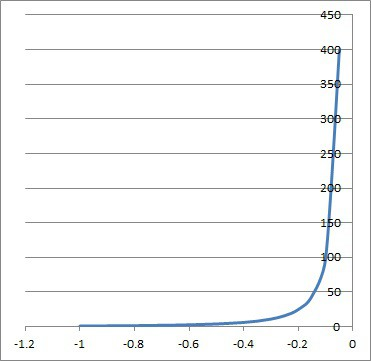The function f(x) = 1 / x2 near x = 0. There is a vertical asymptote at x = 0, since the function grows without bound as x approaches zero.

This is because the function 1 / x2 grows without bound as x approaches zero.

As x approaches zero, x2 remains positive but becomes smaller and smaller.  Since x2 is in the denominator, we will see 1 / x2 increase without bound.

Thus, the left hand limit does not exist in this case (or we could say that the limit is infinity).

### Right Hand Limit Does Not Exist

Just as a left hand limit can fail to exist, a right hand limit can also fail to exist.  Here are some examples where the right hand limit does not exist.

#### Example 1: Right Hand Limit Does Not Exist (Oscillating Values)

Consider the function f(x) = cos(1 / x2).  If we take a right hand limit as x approaches zero:

• Limx->0+f(x)

we will find that the limit does not exist.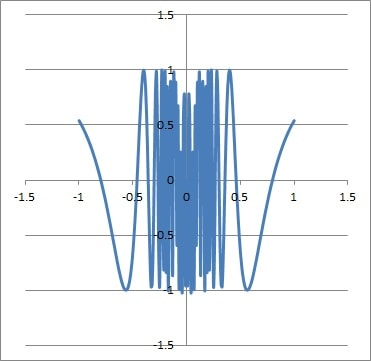The graph of the function f(x) = cos(1 / x2) near x = 0. It oscillates from -1 to 1 and does not settle down to a single value.

This is because the cosine function oscillates between all values from -1 to 1 as x increases.Just like sine, the cosine function continues to oscillate as x increases. It never settles down to a single value.

As x approaches zero, 1 / x2 increases without bound.  So, the limit will never “settle down” to a single value, and will continue oscillating forever.

Thus, the right hand limit does not exist in this case.

#### Example 2: Right Hand Limit Does Not Exist (Vertical Asymptote)

Consider the function f(x) = 1 / (x – 2).  If we take a left hand limit as x approaches 2:

• Limx->2+f(x)

we will find that the limit does not exist.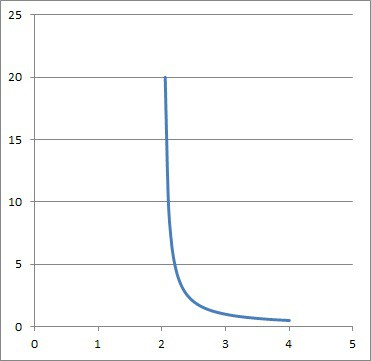The function f(x) = 1 / (x – 2) near x = 2. There is a vertical asymptote at x = 2, since the function grows without bound as x approaches 2.

This is because the function 1 / (x – 2) grows without bound as x approaches 2.

As x approaches 2, (x– 2) approaches zero (and remains positive).  Since (x – 2) is in the denominator, we will see 1 / (x – 2) increase without bound.

Thus, the right hand limit does not exist in this case (or we could say that the limit is infinity).

Having math trouble?

Looking for a tutor?

### Left & Right Hand Limits Both Exist, But They Have Different Values

In some cases, both the left and right hand limits will exist for a function, but they will have different values.  This is sometimes called a “jump” discontinuity.

Here are some cases where the left and right hand limits do not agree.

#### Example 1: Left & Right Hand Limits Both Exist, But They Have Different Values (Absolute Value)

Consider the function f(x) = |x| / x.

If we take a left hand limit as x approaches 0:

• Limx->0-f(x)

we will find that the limit is -1.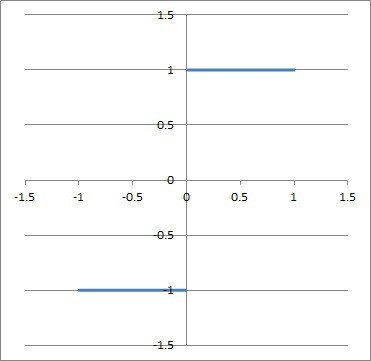The function f(x) = |x| / x. It has a value of 1 for x > 0 and -1 for x < 0 (and is undefined at x = 0). The left and right hand limits exist, but they have different values, so they do not agree.

This is because the numerator and denominator will have opposite signs, but the same absolute value (as you can see in the table below).

Similarly, if we take a right hand limit as x approaches 0:

• Limx->0+f(x)

we will find that the limit is +1.  This is because the numerator and denominator will have the same sign and the same absolute value (as you can see in the table below).

So, the left hand limit (-1) and right hand limit (+1) both exist.  However, they disagree, since they do not have the same value.

Thus, the limit as x approaches zero does not exist for this function.

• Limx->0f(x): DNE (does not exist)

#### Example 2: Left & Right Hand Limits Both Exist, But They Have Different Values (Piecewise)

Consider the following piecewise function: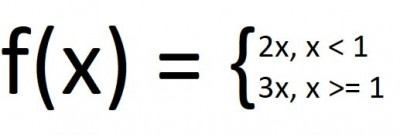This is the definition of a piecewise function with left and right side limits at x = 1 that exist, but do not agree (they have different values).

If we take a left hand limit as x approaches 1:

• Limx->1-f(x)

we will find that the limit is 2.

Similarly, if we take a right hand limit as x approaches 1:

• Limx->1+f(x)

we will find that the limit is 3.

So, the left hand limit (2) and right hand limit (3) both exist.  However, they disagree, since they do not have the same value.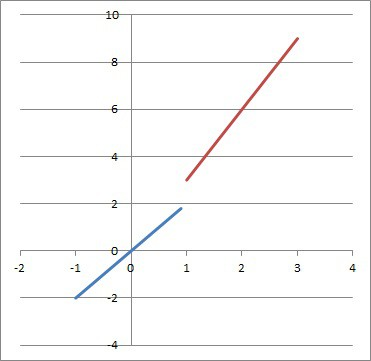The graph of the piecewise function. Notice that the limits as x approach 1 do not agree.

Thus, the limit as x approaches 1 does not exist for this function.

### Function Is Not Defined Due To Domain Restriction

A limit can also fail to exist if a function is not defined due to a domain restriction.

#### Example: Function Is Not Defined Due To Domain Restriction (Square Root)

Consider the function f(x) = √x.

If we take a right hand limit as x approaches 0:

• Limx->0+f(x)

the limit is defined, and the value is 0 (since the square root of zero approaches zero).

However, if we take a left hand limit as x approaches 0:

• Limx->0-f(x)

the limit is undefined in the real numbers.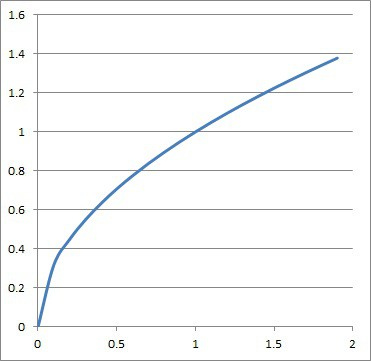The graph of the function f(x) = √x. It is not defined in the real numbers for x < 0.

The reason is that when we approach zero from the left, we are looking at negative values of x.  No matter how small these values become, they are still negative.

The square root of any negative number is an imaginary number, so the limit does not exist.  Thus, the domain restriction for the square root function (x >= 0) means that we cannot take a left hand limit.

Since the left hand limit at zero does not exist, then the limit at zero does not exist for this function.

## What Does It Mean For A Limit To Not Exist?

When the limit of a function does not exist at a point x = c, it means that:

• the function is not continuous at x = c (since there is an oscillating value, vertical asymptote, jump discontinuity, etc.)
• the function is not differentiable at x = c (since a function cannot be differentiable if it is not continuous).

In other words, the existence of a limit for a function at a point is a prerequisite for continuity of the function at that point, which is in turn a prerequisite for differentiability of the function at that point.

## Conclusion

Now you know about the cases when a limit does not exist and what to look for (oscillating values, vertical asymptotes, and jump discontinuities).

If you have the limit of a quotient that results in an indeterminate form (such as 0/0 or infinity/infinity), you can use L’Hopital’s Rule.

You can learn about the floor function (a type of piecewise function) here.